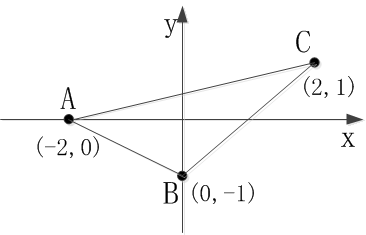# Java开发笔记（二十一）二维数组的扩展

1、利用语句“new 变量类型[顶点数量][方向数量]”分配空间，比如三角形triangle有三个顶点，每个顶点由横纵两个坐标方向组成，则可通过下面这行语句实现：

		// 在两对方括号内分别填入数字，表示数组有多少行多少列
triangle = new double;


2、在分配存储空间的时候立即对数组进行初始化赋值，此时方括号中间不填数字，而在方括号后面添加花括号，并且花括号内部是以逗号分隔的几个一维数组。此时初始化赋值的代码如下所示：

		// 方括号内留空，然后紧跟花括号，花括号内部是以逗号分隔的几个一维数组
double[][] triangle = new double[][]{
new double[]{-2.0, 0.0},
new double[]{0.0, -1.0},
new double[]{2.0, 1.0}
};


3、可见上面的第二种写法实在啰嗦，完全可以参照一维数组的简化写法，把多余的“new double***”统统去掉，于是整个初始化代码精简如下：

		// 赋值等号右边直接跟着花括号，花括号又内嵌好几个花括号分别表示对应的一维数组
double[][] triangle = { {-2.0, 0.0}, {0.0, -1.0}, {2.0, 1.0} };


		// 以下是声明二维数组的第一种形式：“变量类型 数组名称[][]”
double triangle[][];
// 以下是分配二维数组空间的第一种形式
// 在两对方括号内分别填入数字，表示数组有多少行多少列
triangle = new double;
// 数组名称后面的“[数字1][数字2]”，就是数组元素的行列下标，表示当前操作的是第几行第几列的数组元素
triangle = -2.0;
triangle = 0.0;
triangle = 0.0;
triangle = -1.0;
triangle = 2.0;
triangle = 1.0;
// 下面通过循环语句依次读出数组中的所有元素。
// “二维数组名称.length”表示获取该数组的行数
for (int i=0; i<triangle.length; i++) {
// “triangle[i].length”表示获取该数组第i行的列数
for (int j=0; j<triangle[i].length; j++) {
// 打印第i行第j列的数组元素
System.out.println("triangle["+i+"]["+j+"]="+triangle[i][j]);
}
}// 下面通过循环语句依次计算三角形每条边的长度
// 假设第一个数组元素代表点A，第二个数组元素代表点B，第三个数组元素代表点C，
// 则本循环将依次求得AB、AC、BC这三条边的长度
for (int i=0; i<triangle.length-1; i++) {
for (int j=i+1; j<triangle.length; j++) {
// 获取两个顶点在横轴方向的距离
double xDistance = Math.abs(triangle[j] - triangle[i]);
// 获取两个顶点在纵轴方向的距离
double yDistance = Math.abs(triangle[j] - triangle[i]);
// 根据勾股定理计算连接两顶点的斜边长度
double distance = Math.sqrt(xDistance*xDistance + yDistance*yDistance);
System.out.println("i="+i+",j="+j+",distance="+distance);
}
}


i=0,j=1,distance=2.23606797749979
i=0,j=2,distance=4.123105625617661
i=1,j=2,distance=2.8284271247461903


posted @ 2018-11-09 23:01  pinlantu  阅读(1561)  评论(0编辑  收藏  举报